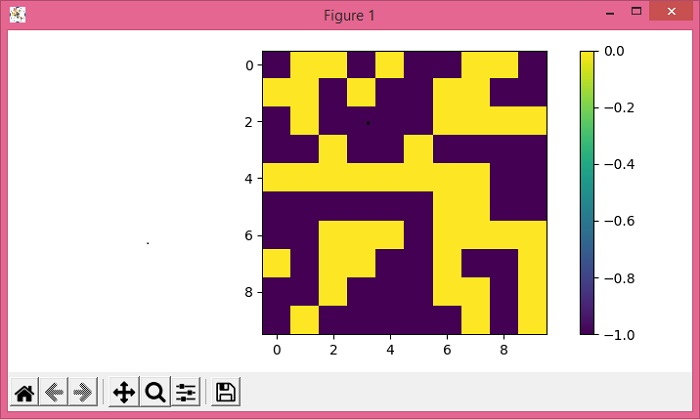# How to retrieve colorbar instance from figure in Matplotlib?

To retrieve colorbar instance from figure in matplotlib, we can use imshow scalar mappable object in colorbar to retrieve colorbar instance.

## Steps

• Get random data with 10×10 dimension of array, data points between -1 to 1.

• Use imshow() method to display data as an image, i.e., on a 2D regular raster.

• Create a colorbar for a ScalarMappable instance, *mappable*, with imshow() object.

• To display the figure, use show() method.

## Example

import numpy as np
from matplotlib import pyplot as plt
plt.rcParams["figure.figsize"] = [7.00, 3.50]
plt.rcParams["figure.autolayout"] = True
data = np.random.randint(-1, 1, (10, 10))
im = plt.imshow(data, interpolation="nearest")
cbar = plt.colorbar(im)
plt.show()

## Output